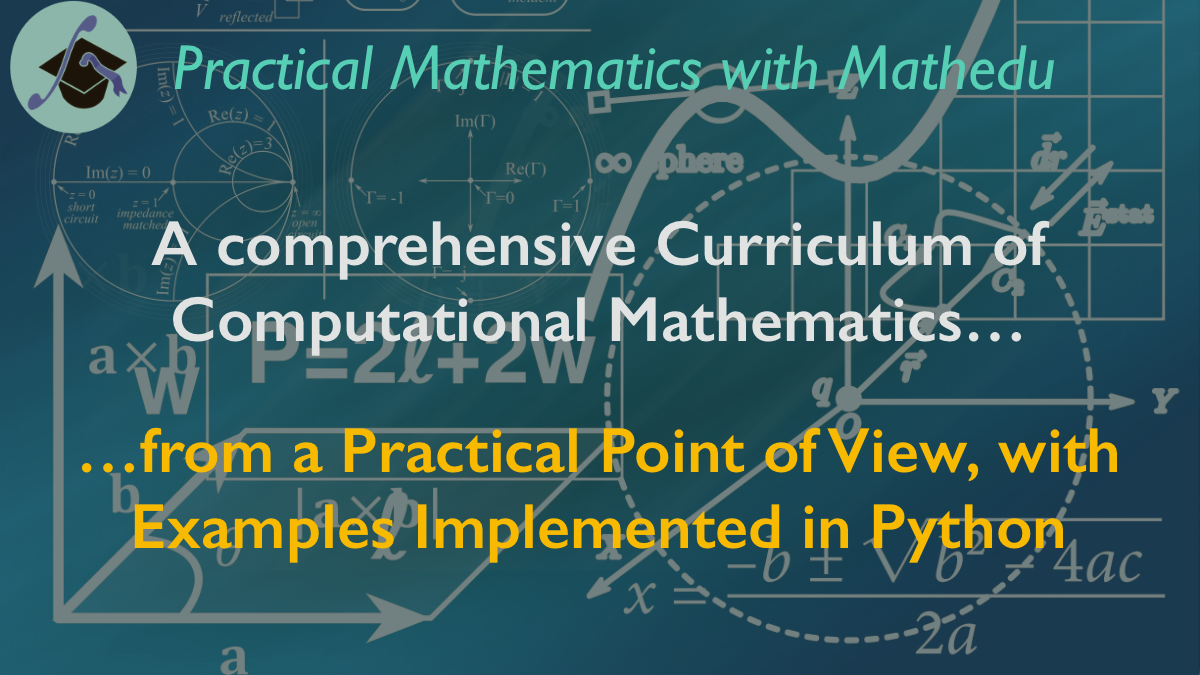# MatheduReengineering MathematicsScheduleNatural Integers MasterClass

AvailableThe Signed Integers MasterClass

April 30, 2021The Fractions and the Rational numbers MasterClass

August 31 2021The Real Numbers and Approximation MasterClass

December 25 2021The Systems of Linear Equations

August 31, 2022The Polynomials and Polynomial Approximations

April 30, 2023The Calculus Classes

2023-2024

More Numerical Analysis

2024, 2025 and beyond…

• Natural Integers Masterclass

### Mastering the construction and manipulation of the Natural integers

• Count and construct the natural integers set
• Prove theorems by recusion, a powerful method of proof
• Order the natural integers set
• Add, subtract and multiply the natural integers
• Powers of 10 and scientific notation
• Exponentiation of the natural integers
• Comparison and multiplication/exponentiation

And the course includes a final project: the combinatory binomial coefficients and the binomiel theorem.

That course includes:

• 54 high quality video lectures,
• 10 downloadable recapitulative documents, one per section, with all the proofs.
• 10 intermidiate tess, one per section, with a process to have them corrected
• One final assessment, to submit to correction and grading

And when the final assessment is successfully passed, the students will get a module cerificate.

### The ordered semi-ring (N,+,x,≤)

• The Signed Integers MasterClass

### Mastering the computation with signed integers

• Discover the negative numbers
• Construct the two-sided integer line of integers Z
• Compare and order integers of any signs
• Add , subtract and multiply integers of any signs
• Discover integer polynomialls
• Exponentiate integers of any sign
• Divide non-zeros integers when integer division is possible
• Practice the signs rules for inequalities
• Discover calculation modulo a positive integer p, and the quotient set Z/pZ

And the course includes a final project: Final Project: The Bezout Identity to calculate the multiplicative inverses in the finite field Z/pZ, with its implementation in Python, and its proof in a downloadable document.

That course includes:

• 58 high quality video lectures,
• 10 downloadable recapitulative documents, one per section, with all the proofs.
• 10 intermidiate tess, one per section, with a process to have them corrected
• One final assessment, to submit to correction and grading

And when the final assessment is successfully passed, the students will get a module cerificate.

### Construct and order the Integers set Z

A free version of that section: Construct and compare the Signed Integers, is scheduled for JJanuary 2021

### Final Project: The Bezout Identity and the finite fields Z/pZ

• The Fractions and the Rational numbers MasterClass

### Mastering the Fractions and the Rational Numbers Construction and Manipulation

In that course, we will discover the fractions of integers, that are interesting by themselves, and the rational numbers, that are represented by fraction, but not reduced to fractions.

This is because many fractions (an infinity in fact) reprent the same rational number.

We willl learn to add, subtract, multiply, divide and exponentiate fractions, and also rational numbers, that are families of fractions.

• The Real Numbers and Approximation MasterClass

### Mastering the Real Numbers Construction and Manipulation

In that course, we will construct the real numbers set as famiies of sequences of rational numbers, the sort of sequences called Cauchy sequences.

(The Cauchy sequences areinteresting by themselves, because they give a rigorous frame to the approximation matter.)

The addition, subtraction, multiplication, division, and exponentiation of real numbers will be comprehensively taught.

• The Calculus Classes

### Mastering Calculus, from a numerical analysis point of view

With the real numbers set and the approximation tools, we shall be ready for the Calculus Classes:

• The systems of linear equations
• The polynomial functions
• The continuity of functions
• The diffenrentiation of functions
• The integration of functions

The particularity of these Calculus classes is that they don't only start from a practical point of view, as all our courses, but it leads also to numerical analysis, the science of algorithms.

So that you will be able to practice immediatly in Python, and to use your mastery for any scientfic computing project you may havr to develop.

• More Numerical Analysis

### Mastering Numerical Analysis of any kind

By the end of the year 2024, you will get more classes of numerical analysis:

• Sequences and series of real numbers
• with the definition of exponential and logarithm functions
• Complex numbers with their exponential
• The polar coordinates in the plane
• The fourier transforms
• The multivariables functions with the optimisation problems
• Eigenvalues and Eigenvectors
• With the conditioning of a problem
• Differential equations of one real variable
• Two boundary values problems